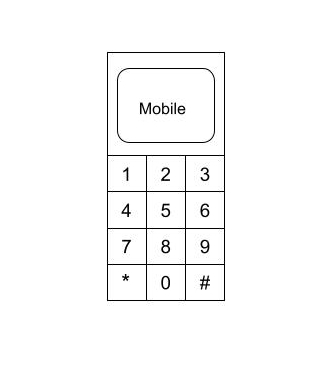3

# Mike and Mobile

Difficulty: MEDIUMContributed By
Himanshu Tanwar
Avg. time to solve
35 min
Success Rate
45%

Problem Statement

#### Mike is a little boy who loves solving math problems. One day he was playing with his mom’s mobile. The mobile keypad contains 12 buttons { 10 digits(0-9) and 2 characters(‘*’ and ‘#’) }. Mike wants to know how many different numbers he can generate after pressing exactly the 'N' buttons on the keypad. Mike presses the buttons with the following rules:

``````1. He always presses the button which has a digit written on it, i.e., he never presses the ‘*’ and ‘#’ button.
2. Once he presses a button, the next button he presses should either be the same button or the button which is adjacent to the previous button.
3. In starting he can press any button except ‘*’ and ‘#’.
``````#### Mike is too little to solve this problem. Help Mike to solve this problem. As the answer can be large, so find the answer modulo 10^9 + 7.

##### Input format :
``````The first line of input contains a single integer 'T', representing the number of test cases or queries to be run.

Then the 'T' test cases follow.

The first and only line of each test case contains a positive integer N, which represents the number of buttons to press.
``````
##### Output Format :
``````For each test case, print the number of numbers that can be generated after pressing exactly the N buttons on the keypad.

The output of each test case is printed in a separate line.
``````
##### Note:
``````You do not need to print anything, it has already been taken care of. Just implement the given function.
``````
##### Constraints :
``````1 <= T <= 10
1 <= N <= 5 * 10^4

Time Limit: 1 sec
``````
##### Sample Input 1:
``````2
1
5
``````
##### Sample Output 1:
``````10
2062
``````
##### Explanation for Sample Input 1:
``````In the 1st test case, Mike can generate the following numbers {0, 1, 2, 3, 4, 5, 6, 7, 8, 9}.

In the 2nd test case, some of the numbers that Mike can generate are {12365, 11111, 74747, 08521,....}.
``````
##### Sample Input 2:
``````2
2
3
``````
##### Sample Output 2:
``````36
138
``````Console